In :
import keras
keras.__version__

Using TensorFlow backend.

Out:
'2.0.8'

# Introduction to generative adversarial networks¶

This notebook contains the second code sample found in Chapter 8, Section 5 of Deep Learning with Python. Note that the original text features far more content, in particular further explanations and figures: in this notebook, you will only find source code and related comments.

[...]

## A schematic GAN implementation¶

In what follows, we explain how to implement a GAN in Keras, in its barest form -- since GANs are quite advanced, diving deeply into the technical details would be out of scope for us. Our specific implementation will be a deep convolutional GAN, or DCGAN: a GAN where the generator and discriminator are deep convnets. In particular, it leverages a Conv2DTranspose layer for image upsampling in the generator.

We will train our GAN on images from CIFAR10, a dataset of 50,000 32x32 RGB images belong to 10 classes (5,000 images per class). To make things even easier, we will only use images belonging to the class "frog".

Schematically, our GAN looks like this:

• A generator network maps vectors of shape (latent_dim,) to images of shape (32, 32, 3).
• A discriminator network maps images of shape (32, 32, 3) to a binary score estimating the probability that the image is real.
• A gan network chains the generator and the discriminator together: gan(x) = discriminator(generator(x)). Thus this gan network maps latent space vectors to the discriminator's assessment of the realism of these latent vectors as decoded by the generator.
• We train the discriminator using examples of real and fake images along with "real"/"fake" labels, as we would train any regular image classification model.
• To train the generator, we use the gradients of the generator's weights with regard to the loss of the gan model. This means that, at every step, we move the weights of the generator in a direction that will make the discriminator more likely to classify as "real" the images decoded by the generator. I.e. we train the generator to fool the discriminator.

## A bag of tricks¶

Training GANs and tuning GAN implementations is notoriously difficult. There are a number of known "tricks" that one should keep in mind. Like most things in deep learning, it is more alchemy than science: these tricks are really just heuristics, not theory-backed guidelines. They are backed by some level of intuitive understanding of the phenomenon at hand, and they are known to work well empirically, albeit not necessarily in every context.

Here are a few of the tricks that we leverage in our own implementation of a GAN generator and discriminator below. It is not an exhaustive list of GAN-related tricks; you will find many more across the GAN literature.

• We use tanh as the last activation in the generator, instead of sigmoid, which would be more commonly found in other types of models.
• We sample points from the latent space using a normal distribution (Gaussian distribution), not a uniform distribution.
• Stochasticity is good to induce robustness. Since GAN training results in a dynamic equilibrium, GANs are likely to get "stuck" in all sorts of ways. Introducing randomness during training helps prevent this. We introduce randomness in two ways: 1) we use dropout in the discriminator, 2) we add some random noise to the labels for the discriminator.
• Sparse gradients can hinder GAN training. In deep learning, sparsity is often a desirable property, but not in GANs. There are two things that can induce gradient sparsity: 1) max pooling operations, 2) ReLU activations. Instead of max pooling, we recommend using strided convolutions for downsampling, and we recommend using a LeakyReLU layer instead of a ReLU activation. It is similar to ReLU but it relaxes sparsity constraints by allowing small negative activation values.
• In generated images, it is common to see "checkerboard artifacts" caused by unequal coverage of the pixel space in the generator. To fix this, we use a kernel size that is divisible by the stride size, whenever we use a strided Conv2DTranpose or Conv2D in both the generator and discriminator.

## The generator¶

First, we develop a generator model, which turns a vector (from the latent space -- during training it will sampled at random) into a candidate image. One of the many issues that commonly arise with GANs is that the generator gets stuck with generated images that look like noise. A possible solution is to use dropout on both the discriminator and generator.

In :
import keras
from keras import layers
import numpy as np

latent_dim = 32
height = 32
width = 32
channels = 3

generator_input = keras.Input(shape=(latent_dim,))

# First, transform the input into a 16x16 128-channels feature map
x = layers.Dense(128 * 16 * 16)(generator_input)
x = layers.LeakyReLU()(x)
x = layers.Reshape((16, 16, 128))(x)

# Then, add a convolution layer
x = layers.LeakyReLU()(x)

# Upsample to 32x32
x = layers.Conv2DTranspose(256, 4, strides=2, padding='same')(x)
x = layers.LeakyReLU()(x)

# Few more conv layers
x = layers.LeakyReLU()(x)
x = layers.LeakyReLU()(x)

# Produce a 32x32 1-channel feature map
x = layers.Conv2D(channels, 7, activation='tanh', padding='same')(x)
generator = keras.models.Model(generator_input, x)
generator.summary()

Using TensorFlow backend.

_________________________________________________________________
Layer (type)                 Output Shape              Param #
=================================================================
input_1 (InputLayer)         (None, 32)                0
_________________________________________________________________
dense_1 (Dense)              (None, 32768)             1081344
_________________________________________________________________
leaky_re_lu_1 (LeakyReLU)    (None, 32768)             0
_________________________________________________________________
reshape_1 (Reshape)          (None, 16, 16, 128)       0
_________________________________________________________________
conv2d_1 (Conv2D)            (None, 16, 16, 256)       819456
_________________________________________________________________
leaky_re_lu_2 (LeakyReLU)    (None, 16, 16, 256)       0
_________________________________________________________________
conv2d_transpose_1 (Conv2DTr (None, 32, 32, 256)       1048832
_________________________________________________________________
leaky_re_lu_3 (LeakyReLU)    (None, 32, 32, 256)       0
_________________________________________________________________
conv2d_2 (Conv2D)            (None, 32, 32, 256)       1638656
_________________________________________________________________
leaky_re_lu_4 (LeakyReLU)    (None, 32, 32, 256)       0
_________________________________________________________________
conv2d_3 (Conv2D)            (None, 32, 32, 256)       1638656
_________________________________________________________________
leaky_re_lu_5 (LeakyReLU)    (None, 32, 32, 256)       0
_________________________________________________________________
conv2d_4 (Conv2D)            (None, 32, 32, 3)         37635
=================================================================
Total params: 6,264,579
Trainable params: 6,264,579
Non-trainable params: 0
_________________________________________________________________


## The discriminator¶

Then, we develop a discriminator model, that takes as input a candidate image (real or synthetic) and classifies it into one of two classes, either "generated image" or "real image that comes from the training set".

In :
discriminator_input = layers.Input(shape=(height, width, channels))
x = layers.Conv2D(128, 3)(discriminator_input)
x = layers.LeakyReLU()(x)
x = layers.Conv2D(128, 4, strides=2)(x)
x = layers.LeakyReLU()(x)
x = layers.Conv2D(128, 4, strides=2)(x)
x = layers.LeakyReLU()(x)
x = layers.Conv2D(128, 4, strides=2)(x)
x = layers.LeakyReLU()(x)
x = layers.Flatten()(x)

# One dropout layer - important trick!
x = layers.Dropout(0.4)(x)

# Classification layer
x = layers.Dense(1, activation='sigmoid')(x)

discriminator = keras.models.Model(discriminator_input, x)
discriminator.summary()

# To stabilize training, we use learning rate decay
# and gradient clipping (by value) in the optimizer.
discriminator_optimizer = keras.optimizers.RMSprop(lr=0.0008, clipvalue=1.0, decay=1e-8)
discriminator.compile(optimizer=discriminator_optimizer, loss='binary_crossentropy')

_________________________________________________________________
Layer (type)                 Output Shape              Param #
=================================================================
input_2 (InputLayer)         (None, 32, 32, 3)         0
_________________________________________________________________
conv2d_5 (Conv2D)            (None, 30, 30, 128)       3584
_________________________________________________________________
leaky_re_lu_6 (LeakyReLU)    (None, 30, 30, 128)       0
_________________________________________________________________
conv2d_6 (Conv2D)            (None, 14, 14, 128)       262272
_________________________________________________________________
leaky_re_lu_7 (LeakyReLU)    (None, 14, 14, 128)       0
_________________________________________________________________
conv2d_7 (Conv2D)            (None, 6, 6, 128)         262272
_________________________________________________________________
leaky_re_lu_8 (LeakyReLU)    (None, 6, 6, 128)         0
_________________________________________________________________
conv2d_8 (Conv2D)            (None, 2, 2, 128)         262272
_________________________________________________________________
leaky_re_lu_9 (LeakyReLU)    (None, 2, 2, 128)         0
_________________________________________________________________
flatten_1 (Flatten)          (None, 512)               0
_________________________________________________________________
dropout_1 (Dropout)          (None, 512)               0
_________________________________________________________________
dense_2 (Dense)              (None, 1)                 513
=================================================================
Total params: 790,913
Trainable params: 790,913
Non-trainable params: 0
_________________________________________________________________


Finally, we setup the GAN, which chains the generator and the discriminator. This is the model that, when trained, will move the generator in a direction that improves its ability to fool the discriminator. This model turns latent space points into a classification decision, "fake" or "real", and it is meant to be trained with labels that are always "these are real images". So training gan will updates the weights of generator in a way that makes discriminator more likely to predict "real" when looking at fake images. Very importantly, we set the discriminator to be frozen during training (non-trainable): its weights will not be updated when training gan. If the discriminator weights could be updated during this process, then we would be training the discriminator to always predict "real", which is not what we want!

In :
# Set discriminator weights to non-trainable
# (will only apply to the gan model)
discriminator.trainable = False

gan_input = keras.Input(shape=(latent_dim,))
gan_output = discriminator(generator(gan_input))
gan = keras.models.Model(gan_input, gan_output)

gan_optimizer = keras.optimizers.RMSprop(lr=0.0004, clipvalue=1.0, decay=1e-8)
gan.compile(optimizer=gan_optimizer, loss='binary_crossentropy')


## How to train your DCGAN¶

Now we can start training. To recapitulate, this is schematically what the training loop looks like:

for each epoch:
* Draw random points in the latent space (random noise).
* Generate images with generator using this random noise.
* Mix the generated images with real ones.
* Train discriminator using these mixed images, with corresponding targets, either "real" (for the real images) or "fake" (for the generated images).
* Draw new random points in the latent space.
* Train gan using these random vectors, with targets that all say "these are real images". This will update the weights of the generator (only, since discriminator is frozen inside gan) to move them towards getting the discriminator to predict "these are real images" for generated images, i.e. this trains the generator to fool the discriminator.

Let's implement it:

In :
import os
from keras.preprocessing import image

(x_train, y_train), (_, _) = keras.datasets.cifar10.load_data()

# Select frog images (class 6)
x_train = x_train[y_train.flatten() == 6]

# Normalize data
x_train = x_train.reshape(
(x_train.shape,) + (height, width, channels)).astype('float32') / 255.

iterations = 10000
batch_size = 20
save_dir = '/home/ubuntu/gan_images/'

# Start training loop
start = 0
for step in range(iterations):
# Sample random points in the latent space
random_latent_vectors = np.random.normal(size=(batch_size, latent_dim))

# Decode them to fake images
generated_images = generator.predict(random_latent_vectors)

# Combine them with real images
stop = start + batch_size
real_images = x_train[start: stop]
combined_images = np.concatenate([generated_images, real_images])

# Assemble labels discriminating real from fake images
labels = np.concatenate([np.ones((batch_size, 1)),
np.zeros((batch_size, 1))])
# Add random noise to the labels - important trick!
labels += 0.05 * np.random.random(labels.shape)

# Train the discriminator
d_loss = discriminator.train_on_batch(combined_images, labels)

# sample random points in the latent space
random_latent_vectors = np.random.normal(size=(batch_size, latent_dim))

# Assemble labels that say "all real images"

# Train the generator (via the gan model,
# where the discriminator weights are frozen)

start += batch_size
if start > len(x_train) - batch_size:
start = 0

# Occasionally save / plot
if step % 100 == 0:
# Save model weights
gan.save_weights('gan.h5')

# Print metrics
print('discriminator loss at step %s: %s' % (step, d_loss))
print('adversarial loss at step %s: %s' % (step, a_loss))

# Save one generated image
img = image.array_to_img(generated_images * 255., scale=False)
img.save(os.path.join(save_dir, 'generated_frog' + str(step) + '.png'))

# Save one real image, for comparison
img = image.array_to_img(real_images * 255., scale=False)
img.save(os.path.join(save_dir, 'real_frog' + str(step) + '.png'))

discriminator loss at step 0: 0.685675
adversarial loss at step 0: 0.667591
discriminator loss at step 100: 0.756201
adversarial loss at step 100: 0.820905
discriminator loss at step 200: 0.699047
adversarial loss at step 200: 0.776581
discriminator loss at step 300: 0.684602
adversarial loss at step 300: 0.513813
discriminator loss at step 400: 0.707092
adversarial loss at step 400: 0.716778
discriminator loss at step 500: 0.686278
adversarial loss at step 500: 0.741214
discriminator loss at step 600: 0.692786
adversarial loss at step 600: 0.745891
discriminator loss at step 700: 0.69771
adversarial loss at step 700: 0.781026
discriminator loss at step 800: 0.69236
adversarial loss at step 800: 0.748769
discriminator loss at step 900: 0.663193
adversarial loss at step 900: 0.689923
discriminator loss at step 1000: 0.706922
adversarial loss at step 1000: 0.741314
discriminator loss at step 1100: 0.682189
adversarial loss at step 1100: 0.76548
discriminator loss at step 1200: 0.687244
adversarial loss at step 1200: 0.746018
discriminator loss at step 1300: 0.697884
adversarial loss at step 1300: 0.766032
discriminator loss at step 1400: 0.691977
adversarial loss at step 1400: 0.735184
discriminator loss at step 1500: 0.696238
adversarial loss at step 1500: 0.738426
discriminator loss at step 1600: 0.698334
adversarial loss at step 1600: 0.741093
discriminator loss at step 1700: 0.70315
adversarial loss at step 1700: 0.736702
discriminator loss at step 1800: 0.693836
adversarial loss at step 1800: 0.742768
discriminator loss at step 1900: 0.69059
adversarial loss at step 1900: 0.741162
discriminator loss at step 2000: 0.696293
adversarial loss at step 2000: 0.755151
discriminator loss at step 2100: 0.686166
adversarial loss at step 2100: 0.755129
discriminator loss at step 2200: 0.692612
adversarial loss at step 2200: 0.772408
discriminator loss at step 2300: 0.704013
adversarial loss at step 2300: 0.776998
discriminator loss at step 2400: 0.693268
adversarial loss at step 2400: 0.70731
discriminator loss at step 2500: 0.684289
adversarial loss at step 2500: 0.742162
discriminator loss at step 2600: 0.700483
adversarial loss at step 2600: 0.734719
discriminator loss at step 2700: 0.699952
adversarial loss at step 2700: 0.759745
discriminator loss at step 2800: 0.697416
adversarial loss at step 2800: 0.733726
discriminator loss at step 2900: 0.697604
adversarial loss at step 2900: 0.740891
discriminator loss at step 3000: 0.698498
adversarial loss at step 3000: 0.754564
discriminator loss at step 3100: 0.695516
adversarial loss at step 3100: 0.759486
discriminator loss at step 3200: 0.693453
adversarial loss at step 3200: 0.769369
discriminator loss at step 3300: 1.5083
adversarial loss at step 3300: 0.726621
discriminator loss at step 3400: 0.686934
adversarial loss at step 3400: 0.747121
discriminator loss at step 3500: 0.689791
adversarial loss at step 3500: 0.751882
discriminator loss at step 3600: 0.71331
adversarial loss at step 3600: 0.704916
discriminator loss at step 3700: 0.690504
adversarial loss at step 3700: 0.853764
discriminator loss at step 3800: 0.688844
adversarial loss at step 3800: 0.791077
discriminator loss at step 3900: 0.679162
adversarial loss at step 3900: 0.724979
discriminator loss at step 4000: 0.676585
adversarial loss at step 4000: 0.69554
discriminator loss at step 4100: 0.693313
adversarial loss at step 4100: 0.742666
discriminator loss at step 4200: 0.678367
adversarial loss at step 4200: 0.778793
discriminator loss at step 4300: 0.699712
adversarial loss at step 4300: 0.740457
discriminator loss at step 4400: 0.697605
adversarial loss at step 4400: 0.755847
discriminator loss at step 4500: 0.710596
adversarial loss at step 4500: 0.814832
discriminator loss at step 4600: 0.706518
adversarial loss at step 4600: 0.83636
discriminator loss at step 4700: 0.687217
adversarial loss at step 4700: 0.775736
discriminator loss at step 4800: 0.769103
adversarial loss at step 4800: 0.774639
discriminator loss at step 4900: 0.692414
adversarial loss at step 4900: 0.775192
discriminator loss at step 5000: 0.715357
adversarial loss at step 5000: 0.775003
discriminator loss at step 5100: 0.703434
adversarial loss at step 5100: 0.940242
discriminator loss at step 5200: 0.704034
adversarial loss at step 5200: 0.708327
discriminator loss at step 5300: 0.698559
adversarial loss at step 5300: 0.730377
discriminator loss at step 5400: 0.684378
adversarial loss at step 5400: 0.759259
discriminator loss at step 5500: 0.693699
adversarial loss at step 5500: 0.700122
discriminator loss at step 5600: 0.715242
adversarial loss at step 5600: 0.808961
discriminator loss at step 5700: 0.689339
adversarial loss at step 5700: 0.621725
discriminator loss at step 5800: 0.679717
adversarial loss at step 5800: 0.787711
discriminator loss at step 5900: 0.700126
adversarial loss at step 5900: 0.742493
discriminator loss at step 6000: 0.692087
adversarial loss at step 6000: 0.839669
discriminator loss at step 6100: 0.677867
adversarial loss at step 6100: 0.797158
discriminator loss at step 6200: 0.70392
adversarial loss at step 6200: 0.842135
discriminator loss at step 6300: 0.688377
adversarial loss at step 6300: 0.718633
discriminator loss at step 6400: 0.781234
adversarial loss at step 6400: 0.710833
discriminator loss at step 6500: 0.682696
adversarial loss at step 6500: 0.739674
discriminator loss at step 6600: 0.693081
adversarial loss at step 6600: 0.747336
discriminator loss at step 6700: 0.681836
adversarial loss at step 6700: 0.780143
discriminator loss at step 6800: 0.728136
adversarial loss at step 6800: 0.838522
discriminator loss at step 6900: 0.660475
adversarial loss at step 6900: 0.717434
discriminator loss at step 7000: 0.672144
adversarial loss at step 7000: 0.948783
discriminator loss at step 7100: 0.692428
adversarial loss at step 7100: 0.837047
discriminator loss at step 7200: 0.731133
adversarial loss at step 7200: 0.728315
discriminator loss at step 7300: 0.671766
adversarial loss at step 7300: 0.793155
discriminator loss at step 7400: 0.712387
adversarial loss at step 7400: 0.807759
discriminator loss at step 7500: 0.68638
adversarial loss at step 7500: 0.967421
discriminator loss at step 7600: 0.690096
adversarial loss at step 7600: 0.811904
discriminator loss at step 7700: 0.702784
adversarial loss at step 7700: 0.867017
discriminator loss at step 7800: 0.674138
adversarial loss at step 7800: 0.837909
discriminator loss at step 7900: 0.674747
adversarial loss at step 7900: 0.743664
discriminator loss at step 8000: 0.680357
adversarial loss at step 8000: 0.810859
discriminator loss at step 8100: 0.688885
adversarial loss at step 8100: 0.786809
discriminator loss at step 8200: 0.671557
adversarial loss at step 8200: 0.784159
discriminator loss at step 8300: 0.70359
adversarial loss at step 8300: 0.95692
discriminator loss at step 8400: 0.720167
adversarial loss at step 8400: 1.14066
discriminator loss at step 8500: 0.747376
adversarial loss at step 8500: 0.630725
discriminator loss at step 8600: 0.688931
adversarial loss at step 8600: 0.849245
discriminator loss at step 8700: 0.707559
adversarial loss at step 8700: 0.713202
discriminator loss at step 8800: 0.673593
adversarial loss at step 8800: 0.832419
discriminator loss at step 8900: 0.6777
adversarial loss at step 8900: 0.773395
discriminator loss at step 9000: 0.659887
adversarial loss at step 9000: 0.77255
discriminator loss at step 9100: 0.675182
adversarial loss at step 9100: 0.749544
discriminator loss at step 9200: 0.687147
adversarial loss at step 9200: 0.836509
discriminator loss at step 9300: 0.690807
adversarial loss at step 9300: 0.829561
discriminator loss at step 9400: 0.656649
adversarial loss at step 9400: 0.788181
discriminator loss at step 9500: 0.703494
adversarial loss at step 9500: 0.78302
discriminator loss at step 9600: 0.680718
adversarial loss at step 9600: 0.813078
discriminator loss at step 9700: 0.704956
adversarial loss at step 9700: 0.761652
discriminator loss at step 9800: 0.673504
adversarial loss at step 9800: 0.853213
discriminator loss at step 9900: 0.669288
adversarial loss at step 9900: 0.677691


Let's display a few of our fake images:

In :
import matplotlib.pyplot as plt

# Sample random points in the latent space
random_latent_vectors = np.random.normal(size=(10, latent_dim))

# Decode them to fake images
generated_images = generator.predict(random_latent_vectors)

for i in range(generated_images.shape):
img = image.array_to_img(generated_images[i] * 255., scale=False)
plt.figure()
plt.imshow(img)

plt.show()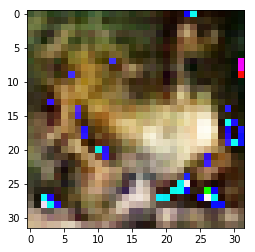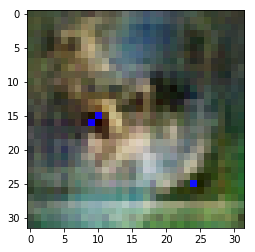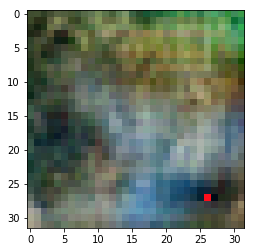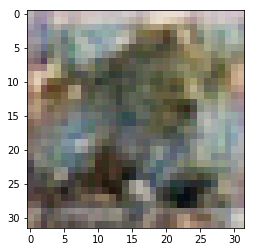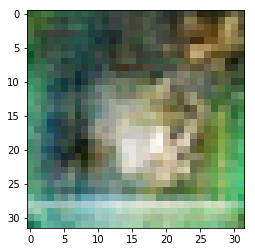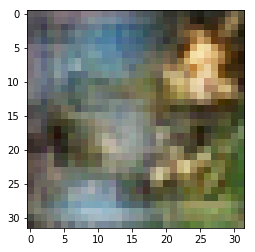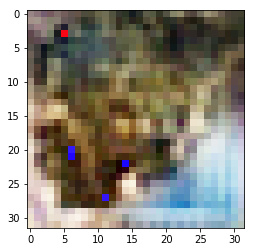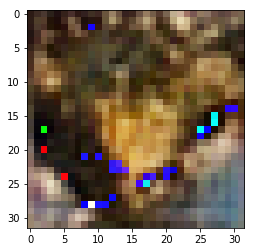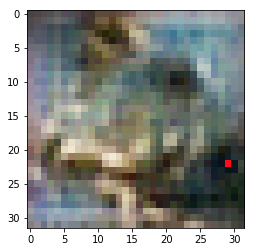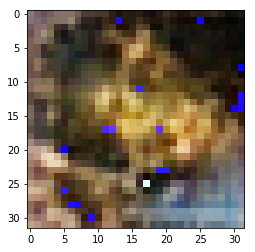Froggy with some pixellated artifacts.